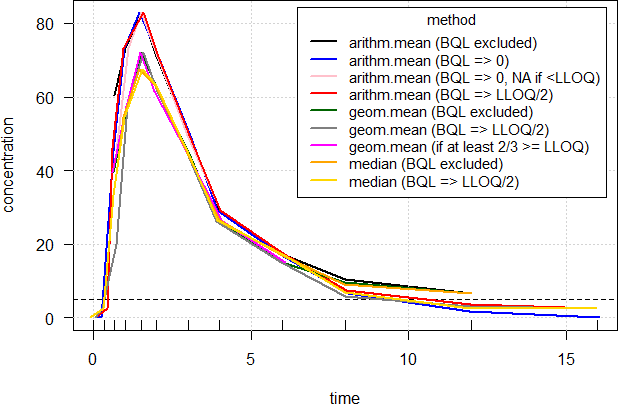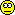## Graphing Mean PK profile [R for BE/BA]

Hi nobody,

» » Some people set all BQLs to zero in order to calculate the arithmetic mean. Others set the first BQL after tmax to LLOQ/2, and, and, and… There will always be a bias.
»
» How would you otherwise calculate the geo. mean profile?

Tons of rules. Mine: If at any given time point ≥⅔ of concentrations are ≥LLOQ I calculate the geometric mean of those (i.e., exclude the BQLs). If less, I don’t calculate the geometric mean at all. Luckily in my studies the LLOQ was always low enough.Had a look at the Canadian guidance and the example given in the appendix. HC calculates the arithmetic (!) mean whilst treating BQLs as zero (Table A1-B/C, p.23/24). If a mean is <LLOQ, it is not shown in the plot (Figure 1, p.31).

Played a bit with the example (after correcting the typo 3723 → 37.23 which sits happily in the table for 25 years). R-code at the end.
                             method  0 0.33  0.67     1   1.5     2     3     4     6     8   12  16          arithm.mean (BQL excluded)  0  NaN 60.44 73.28 82.85 70.94 49.62 29.09 17.19 10.38 6.56 NaN              arithm.mean (BQL => 0)  0  0.0 45.33 73.28 82.85 70.94 49.62 29.09 17.19  6.49 1.64 0.0 arithm.mean (BQL => 0, NA if <LLOQ) NA   NA 45.33 73.28 82.85 70.94 49.62 29.09 17.19  6.49   NA  NA         arithm.mean (BQL => LLOQ/2)  0  2.5 45.95 73.28 82.85 70.94 49.62 29.09 17.19  7.42 3.52 2.5            geom.mean (BQL excluded)  0  NaN 39.58 57.55 71.98 61.44 45.24 26.08 14.98  9.74 6.51 NaN           geom.mean (BQL => LLOQ/2)  0  2.5 19.84 57.55 71.98 61.44 45.24 26.08 14.98  5.85 3.18 2.5 geom.mean (if at least 2/3 >= LLOQ)  0   NA 39.58 57.55 71.98 61.44 45.24 26.08 14.98    NA   NA  NA               median (BQL excluded)  0   NA 40.97 54.39 67.57 63.05 44.69 26.46 16.86  9.19 6.62  NA              median (BQL => LLOQ/2)  0  2.5 33.24 54.39 67.57 63.05 44.69 26.46 16.86  6.84 2.50 2.5Added some jitter to separate the lines.
Now what? I think everything (except arithmetic means) is OK. Coming up with sumfink which is <LLOQ? Hhm. As soon as we have a single value <LLOQ it might be that the estimate is <LLOQ as well (if all others are LLOQ). But how much? No idea.

Will submit a study to HC soon. Will report back how they like my approach.» And AUCtlast is a mess with different tlast for T and R and and and.

Can’t agree more.

» Do a spaghetti plot for T and R and individual T+R for each subject and you might get completely different "ideas" / insights...

Yes and yes. Henning Blume always apologized when presenting (arithmetic) mean plots. I’m pissed when I get a report without spaghetti plots.

loc.stat <- function(x, type, na.rm) {   non.numerics    <- which(is.na(suppressWarnings(as.numeric(x))))   x[non.numerics] <- NA   x <- as.numeric(x)   switch(type,          arith.mean = round(mean(x, na.rm=na.rm), 2),          median     = round(median(x, na.rm=na.rm), 2),          geom.mean  = round(exp(mean(log(x), na.rm=na.rm)), 2)) } t <- c(0, 0.33, 0.67, 1, 1.5, 2, 3, 4, 6, 8, 12, 16) LLOQ <- 5 df <- data.frame(ID=c("A", "B", "C", "E", "F", "G", "H", "I",                       "K", "L", "M", "N", "O", "P", "Q", "R"),                  t.1=rep(0, 16), t.2=rep("BQL", 16),                  t.3=c(116.40,  88.45,  "BQL",  37.23,  29.25,   6.89, 113.50, 181.90,                         42.71,  14.29,   8.21,  47.20,  "BQL",  39.23,  "BQL",  "BQL"),                  t.4=c(124.60, 121.40,  95.57,  37.26,  62.88,  50.04, 218.70, 135.80,                         58.75,  21.32,  48.87,  34.90,  20.35,  86.29,  30.86,  24.84),                  t.5=c(126.20, 206.90, 122.80,  35.90,  64.26,  55.27, 125.80,  96.51,                         59.68,  24.32,  57.05,  34.90,  70.88,  97.46,  88.38,  59.27),                  t.6=c(107.60, 179.00, 103.20,  28.87,  84.67,  51.68,  69.77,  90.50,                         54.37,  25.56,  56.32,  24.19,  70.60,  52.26,  37.67,  98.82),                  t.7=c( 45.65,  84.53, 101.70,  28.48,  45.21,  38.58,  45.03,  62.58,                         44.35,  25.51,  42.08,  20.11,  70.38,  40.53,  29.28,  69.98),                  t.8=c( 33.22,  40.02,  57.65,  25.10,  25.05,  26.19,  32.78,  30.43,                         22.94,  10.49,  24.79,   8.08,  40.51,  26.74,  14.99,  46.50),                  t.9=c( 16.11,  38.01,  23.85,  24.91,  17.18,   7.79,  18.55,  18.50,                         11.58,   5.49,  16.54,   7.27,  26.93,  12.54,   6.38,  23.46),                 t.10=c( 12.60,  15.12,  14.59,   6.72,   8.47,  "BQL",   5.42,  "BQL",                          6.95,  "BQL",  15.81,  "BQL",   8.20,  "BQL",  "BQL",   9.91),                 t.11=c( "BQL",   5.39,   6.29,  "BQL",  "BQL",  "BQL",  "BQL",  "BQL",                         "BQL",  "BQL",   7.60,  "BQL",  "BQL",  "BQL",  "BQL",   6.96),                 t.12=rep("BQL", 16), stringsAsFactors=FALSE) loc <- data.frame(method=c("arithm.mean (BQL excluded)",                            "arithm.mean (BQL => 0)",                            "arithm.mean (BQL => 0, NA if <LLOQ)",                            "arithm.mean (BQL => LLOQ/2)",                            "geom.mean (BQL excluded)",                            "geom.mean (BQL => LLOQ/2)",                            "geom.mean (if at least 2/3 >= LLOQ)",                            "median (BQL excluded)",                            "median (BQL => LLOQ/2)"),                   t.1=NA, t.2=NA, t.3=NA, t.4=NA, t.5=NA, t.6=NA,                   t.7=NA, t.8=NA, t.9=NA, t.10=NA, t.11=NA, t.12=NA,                   stringsAsFactors=FALSE) names(df)[2:13] <- names(loc)[2:13] <- t for (j in 2:ncol(df)) {    x <- df[, j]    loc[1, j] <- loc.stat(x, "arith.mean", TRUE)    y <- x    y[which(x == "BQL")] <- 0    loc[2, j] <- loc.stat(y, "arith.mean", FALSE)    ifelse(loc[2, j] >= LLOQ, loc[3, j] <- loc[2, j], loc[3, j] <- NA)    y <- x    y[which(x == "BQL")] <- LLOQ/2    loc[4, j] <- loc.stat(y, "arith.mean", FALSE)    loc[5, j] <- loc.stat(x, "geom.mean", TRUE)    loc[6, j] <- loc.stat(y, "geom.mean", FALSE)    if (length(which(x != "BQL")) >= nrow(df)*2/3) {       loc[7, j] <- loc[4, j]    } else {       loc[7, j] <- NA    }    loc[8, j] <- loc.stat(x, "median", TRUE)    loc[9, j] <- loc.stat(y, "median", FALSE) } clr <- c("black", "blue", "pink", "red", "darkgreen",          "gray50", "magenta", "orange", "gold") print(df, row.names=FALSE);print(loc, row.names=FALSE) plot(t, loc[1, 2:ncol(df)], type="l", las=1, col=clr, lwd=2,      ylim=c(0, max(loc[, 2:ncol(df)], na.rm=TRUE)),      xlab="time", ylab="concentration") abline(h=LLOQ, lty=2) grid(); rug(t) for (j in 2:9) {   lines(jitter(t, factor=1.5), loc[j, 2:ncol(df)], col=clr[j], lwd=2) } legend("topright", bg="white", inset=0.02, title="method",        legend=loc[, 1], lwd=2, col=clr, cex=0.9)

Cheers,
Helmut SchützThe quality of responses received is directly proportional to the quality of the question asked. 🚮
Science QuotesIng. Helmut Schütz